# 堆排序以及最大优先队列

## 最大堆的性质

1. 是一棵近似的完全二叉树，除了最底层，其它是全满，且从左向右填充。
2. 树的每个节点对应数组一个元素，根节点对应数组下标`0`元素。
3. 对于下标`i`，它的父节点下标为`(i + 1) / 2 - 1`，左孩子节点下标为`i * 2 + 1`，右孩子节点下标为`i * 2 + 2`
4. 最大堆中，每个结点都必须大于等于左右孩子节点。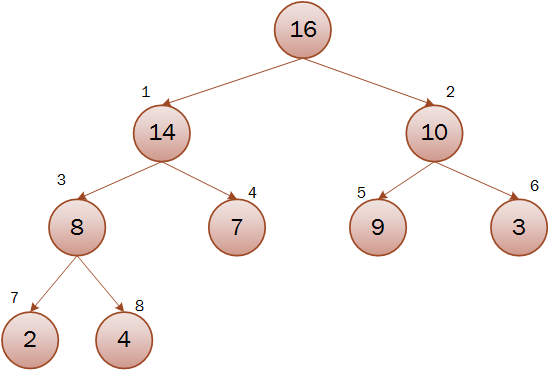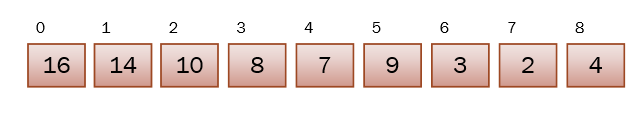## 堆排序

#### 1.维护最大堆

``````private void maxHeapify(int[] a, int i, int length) {
int l = left(i);
int r = right(i);
int max;
if (l < length && a[l] > a[i]) {
max = l;
} else {
max = i;
}
if (r < length && a[r] > a[max]) {
max = r;
}
if (max != i) {
swap(a, i, max);
maxHeapify(a, max, length);
}
}
``````

``````    private int left(int i) {
return i * 2 + 1;
}

private int right(int i) {
return i * 2 + 2;
}
``````

``````    private void swap(int[] a, int i, int j) {
int tmp = a[i];
a[i] = a[j];
a[j] = tmp;
}
``````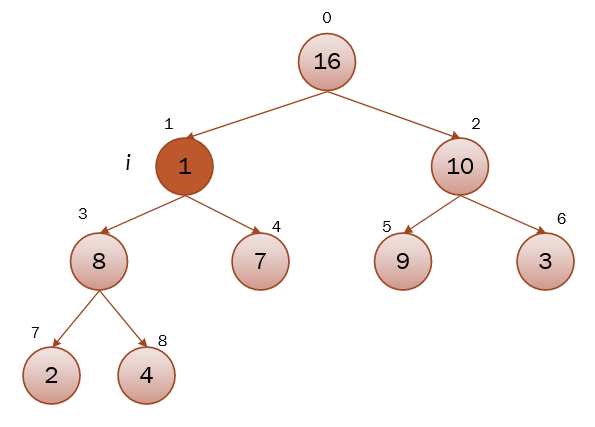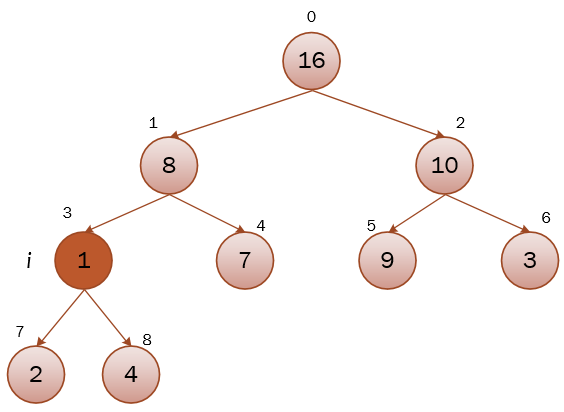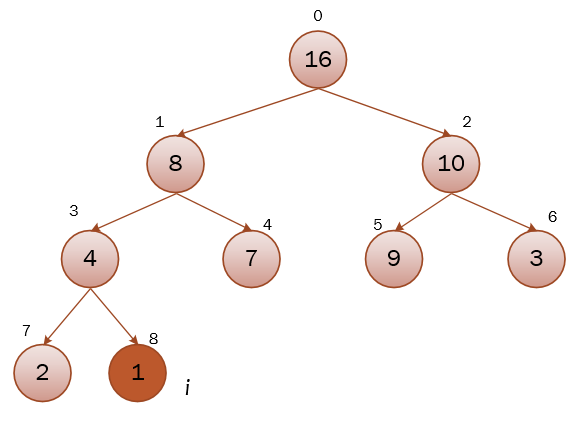#### 2.建立最大堆

``````    private void buildMaxHeap(int a[]) {
for (int i = a.length / 2 + 1; i >= 0; i--) {
maxHeapify(a, i);
}
}
``````#### 3.堆排序

``````    public void heapSort(int[] a) {
buildMaxHeap(a);//首先建立最大堆，完成后第一个元素为最大值
int length = a.length;
for (int i = a.length - 1; i >= 1; i--) {
swap(a, i, 0);//将第一个最大的元素移到后面，并且在maxHeapify的过程中通过减小length忽略它
length--;
maxHeapify(a, 0, length);
}
}
``````

## 最大优先队列

`maximum()`最简单，直接返回第一个元素：

``````    public int maximum() {
return a;
}
``````

`extractMax`也很简单，取出第一个后，只需把最后一个元素放到第一个，然后对第一个元素进行维护最大堆即可：

``````public int extractMax() {
int max = a;
a = a[a.length - 1];
int[] newA = new int[a.length - 1];
System.arraycopy(a, 0, newA, 0, newA.length);
maxHeapify(newA, 0, newA.length);
return max;
}
``````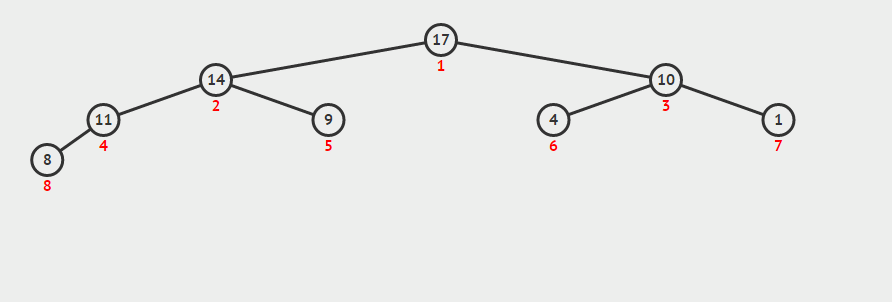`heapIncreaseKey(i, key)`会增大下标为`i`的元素为`key`。首先将`a[i]`的值更新为`key`，因为增大的`a[i]`关键字可能会违背最大堆的性质，因此我们需要对`a[i]`进行`逐级上升`。即将当前元素逐级与父节点比较，如果大于父节点，则与父节点进行交换，一直到当前元素小于父节点为止：

``````public void heapIncreaseKey(int i, int key) {
if (key > a[i]) {
a[i] = key;
while (i > 0 && a[parent(i)] < a[i]) {  //逐级上升
swap(a, parent(i), i);
i = parent(i);
}
} else {
throw new IllegalArgumentException("key is too small");
}
}

private int parent(int i) {
return (i + 1) / 2 - 1;
}
``````

`maxHeapInsert(key)`十分简单，因为它等价于数组长度增加一，然后最后一个元素设置为`－∞`，然后把它增大为`key`的操作：

``````public void maxHeapInsert(int key) {
int[] newA = new int[a.length + 1];
System.arraycopy(a, 0, newA, 0, a.length);
newA[newA.length - 1] = Integer.MIN_VALUE;
this.a = newA;
heapIncreaseKey(a.length - 1, key);
}
``````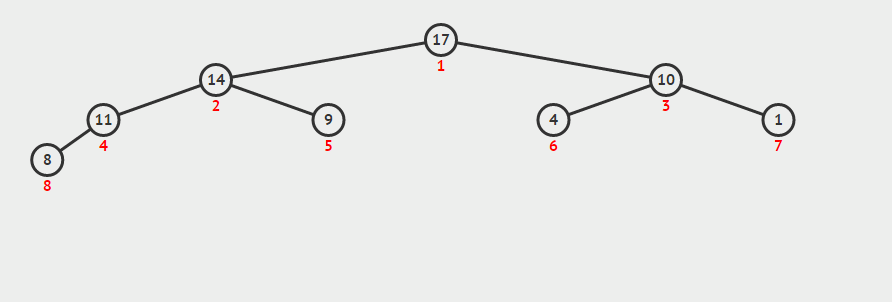This form is protected by reCAPTCHA.